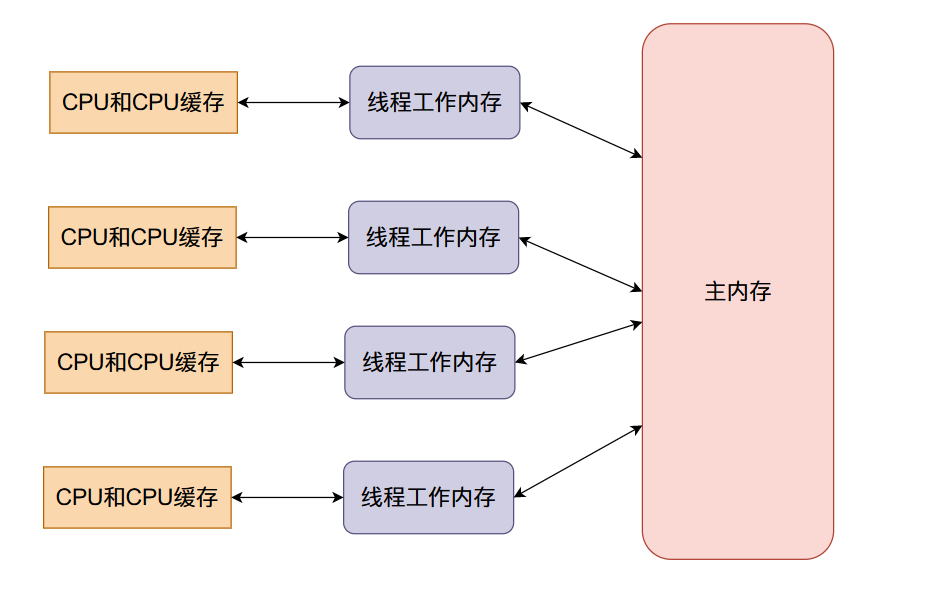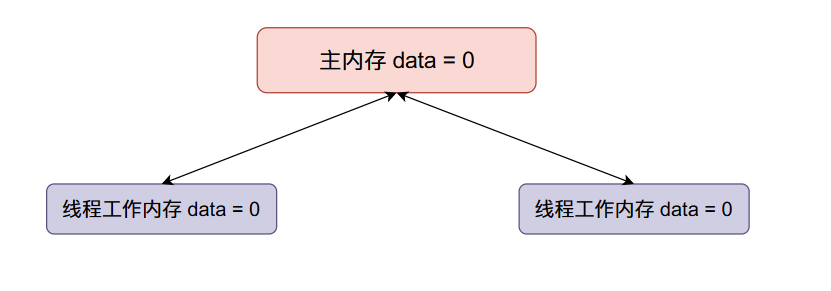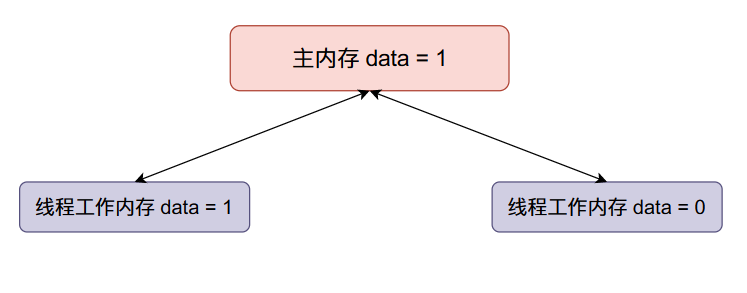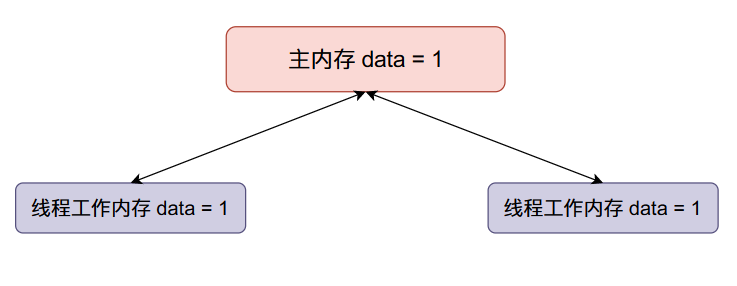# 并发程序的噩梦——数据竞争

## 并发不安全版本

``````public class Sum {

public static int data;

public static void main(String[] args) throws InterruptedException {
for (int i = 0; i < 100000; i++)
data++;
});

for (int i = 0; i < 100000; i++)
data++;
});

t1.start();
t2.start();
// 让主线程等待 t1 和 t2
// 直到 t1 和 t2 执行完成
t1.join();
t2.join();
System.out.println(data);
}
}
// 输出结果
131888
```````data`全局变量保存在主内存当中，当现成开始执行的时候会从主内存拷贝一份到线程的工作内存当中，也就是线程的本地内存，在本地进行计算之后就会将本地内存当中的数据同步到主内存

• 主内存`data`的初始值等于0，两个线程得到的`data`初始值都等于0。• 现在线程一将`data`加一，然后线程一将`data`的值同步回主内存，整个内存的数据变化如下：• 现在线程二`data`加一，然后将`data`的值同步回主内存（将原来主内存的值覆盖掉了）：## 忙等待（Busy waiting）

``````public class BusyWaiting {

public static int sum;
public static boolean flag;

public static void main(String[] args) throws InterruptedException {
for (int i = 0; i < 100000; i++) {
// 当 flag == false 的时候这个线程进行
// sum++ 操作然后让 flat = true
// 让另外一个线程进行 sum++ 操作
while (flag);
sum++;
flag = true;
System.out.println("Thread 1 : " + sum);
}
});

for (int i = 0; i < 100000; i++) {
// 当 flag = true 的之后这个线程进行
// sum++ 操作然后让 flat = false
// 让另外一个线程进行 sum++ 操作
while (!flag) ;
sum++;
flag = false;
System.out.println("Thread 2 : " + sum);
}
});
t1.start();
t2.start();
// 让主线程等待 t1 和 t2
// 直到 t1 和 t2 执行完成
t1.join();
t2.join();
System.out.println(sum);
}
}

``````

## 忙等待中数据竞争的BUG

``````public class BusyWaiting {

public static volatile int sum;
public static volatile boolean flag;

public static void main(String[] args) throws InterruptedException {
for (int i = 0; i < 100000; i++) {
// 当 flag == false 的时候这个线程进行
// sum++ 操作然后让 flat = true
// 让另外一个线程进行 sum++ 操作
while (flag);
sum++;
flag = true;
System.out.println("Thread 1 : " + sum);
}
});

for (int i = 0; i < 100000; i++) {
// 当 flag = true 的之后这个线程进行
// sum++ 操作然后让 flat = false
// 让另外一个线程进行 sum++ 操作
while (!flag) ;
sum++;
flag = false;
System.out.println("Thread 2 : " + sum);
}
});
t1.start();
t2.start();
// 让主线程等待 t1 和 t2
// 直到 t1 和 t2 执行完成
t1.join();
t2.join();
System.out.println(sum);
}
}
``````

## synchronized并发安全版本

`synchronized``java`语言的关键字，它可以用来保证程序的原子性。在并发的情况下我们可以用它来保证我们的程序在某个时刻只能有一个线程执行，保证同一时刻只有一个线程获得`synchronized`锁对象。

``````public class Sum01 {
public static int sum;

public static synchronized void addSum() {
for (int i = 0; i < 100000; i++)
sum++;
}

public static void main(String[] args) throws InterruptedException {

t1.start();
t2.start();
// 让主线程等待 t1 和 t2
// 直到 t1 和 t2 执行完成
t1.join();
t2.join();

System.out.println(sum);
}
}

// 输出结果
200000
``````

``````public class Sum02 {

public static int sum;
public static Object lock = new Object();

for (int i = 0; i < 100000; i++)
synchronized (lock) {
sum++;
}
}

public static void main(String[] args) throws InterruptedException {
t1.start();
t2.start();
// 让主线程等待 t1 和 t2
// 直到 t1 和 t2 执行完成
t1.join();
t2.join();

System.out.println(sum);
}
}
``````

``````// synchronized 修饰的代码块称作同步代码块 ，
// lock 是一个 全局的静态类变量 只有竞争到 lock 对象的线程
// 才能够进入同步代码块 同样的每一个时刻只能有一个线程进入
synchronized (lock) {
sum++;
}
``````

``````public class Sum03 {
public static int sum;
public static Object lock = new Object();

int tempSum = 0;
for (int i = 0; i < 100000; i++)
tempSum++;
synchronized (lock) {
sum += tempSum;
}
}

public static void main(String[] args) throws InterruptedException {
t1.start();
t2.start();
// 让主线程等待 t1 和 t2
// 直到 t1 和 t2 执行完成
t1.join();
t2.join();

System.out.println(sum);
}
}
``````

## 使用锁进行临界区的保护

`java`当中除了使用`synchronized`形成同步方法或者同步代码块的方法保证多个线程访问临界区的顺序，还可以使用锁对临界区进行保护。在`java`当中一个非常常用的锁就是可重入锁`ReentrantLock`，可以保证在`lock``unlock`之间的区域在每一个时刻只有一个线程在执行。

``````import java.util.concurrent.locks.ReentrantLock;

public class Sum04 {

public static int sum;
public static ReentrantLock lock = new ReentrantLock();

int tempSum = 0;
for (int i = 0; i < 100000; i++)
tempSum++;
lock.lock();
try {
sum += tempSum;
}catch (Exception ignored) {

}finally {
lock.unlock();
}
}

public static void main(String[] args) throws InterruptedException {
t1.start();
t2.start();
// 让主线程等待 t1 和 t2
// 直到 t1 和 t2 执行完成
t1.join();
t2.join();

System.out.println(sum);
}
}
``````

## synchronized和锁对可见性的影响

• `synchronized`关键字能够保证可见性，`synchronized `关键字能够不仅能够保证同一时刻只有一个线程获得锁，并且还会确保在锁释放之前，会将对变量的修改刷新到主内存当中。
• `JDK`给我们提供的锁工具（比如`ReentrantLock`）也能够保证可见性，锁的lock方法能够保证在同一时刻只有一个线程获得锁然后执行同步方法，并且会确保在锁释放(锁的unlock方法)之前会将对变量的修改刷新到主内存当中。

## 数据竞争的知名后果——死锁

``````public class DeadLock {

public static Object fork = new Object();
public static Object chopsticks = new Object();

public static void fork() {
synchronized (fork) {
synchronized (chopsticks) {
}
}
}

public static void chopsticks() {
synchronized (chopsticks) {
synchronized (fork) {
}
}
}

public static void main(String[] args) throws InterruptedException {

t1.start();
t2.start();

t1.join();
t2.join();
}
}
// 输出结果
// 在这里已经卡死
``````
• 线程1想先获得叉子，再获得筷子。
• 线程2想先获得筷子，再获得叉子。

### 死锁产生的条件

• 互斥条件：一个资源只能被一个线程占有，如果其他线程想得到这个资源必须由其他线程释放。
• 不剥夺条件：一个线程获得的资源在没有使用完之前不能被其他线程强行获得，只能由线程主动释放。
• 请求保持条件：至少保持了一个资源而且提出新的资源请求，比如上面线程1获得了叉子又请求筷子。
• 循环等待条件：线程1请求线程2的资源，线程2请求线程3的资源，….，线程\(N\)请求线程1的资源。